Courses

# Deflection of Beams Civil Engineering (CE) Notes | EduRev

## Civil Engineering (CE) : Deflection of Beams Civil Engineering (CE) Notes | EduRev

The document Deflection of Beams Civil Engineering (CE) Notes | EduRev is a part of the Civil Engineering (CE) Course Civil Engineering SSC JE (Technical).
All you need of Civil Engineering (CE) at this link: Civil Engineering (CE)

Deflection of Beams

• The amount by which a beam deflects depends upon its cross-section and the bending moment. For modern design there are two governing criteria viz. strength and stiffness.
• As per the strength criterion, the beam should be strong enough to resist bending moment and shear force or in other words beam should be strong enough to resist the bending stresses and shear stresses. And as per the stiffness criterion of the beam design, which is equally important, it should be stiff enough to resist the deflection of the beam or in other words the beam should be stiff enough not to deflect more than the permissible limit. It means serviceability condition must be satisfied.

METHODS FOR SLOPE AND DEFLECTION AT A SECTION
1. Double Integration method

• This method is suitable for simple loading in simply supported beams and cantilevers with uniformly distributed loads and triangular loadings.
•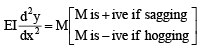After integrating end conditions are applied for determination of constants of integration.

2. Macaulay’s method

• This is a convenient method for beams subjected to point loading or in general discontinuous loads or beams subjected to couples (concentrated moments).
• This method is similar to the double integration method but specialty of this method lies in the manner in which the bending moment at any section is expressed and in the manner in which integration is carried out.

3. Moment Area Method

• This method is suitable for cantilevers and simply supported beams carrying symmetrical loadings and beams fixed at both ends i.e., those beams for which the area and C.G. of area of B.M.D. can be found easily. This means this method is not suitable for triangular loading and irregular loading. This can also be used for non prismatic bars.
• This method is suitable for
(i) Cantilevers (because slope at the fixed end is zero).
(ii) Simply supported beams carrying symmetrical loading (slope at mid span is zero).
(iii) Beams fixed at both ends (slope at each end is zero).

Note: In moment area method continuity of slope is assumed so it is not applied when internal hinges are present.

PROPERTIES OF PLANE AREAS
Notation : A = area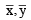= distances of centroid C
Ix, Iy = moments of inertia with respect to the x and y axes respectively.

Ixy = moments of inertia with respect to the x and y axes
Ip = Ix + Iy = polar moment of inertia
IBB = moment of inertia with respect to axis B-B.

(a) Rectangle (Origin of axes at centroid)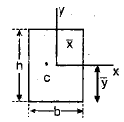A = bh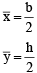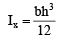Ixy = 0

(b) Rectangle (Origin of axes at corner)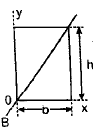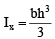(c) Triangle (Origin of axes at centroid).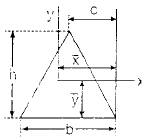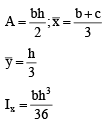(d) Triangle (Origin of axes at vertex).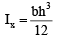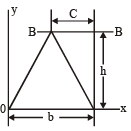(e) Isosceles triangle (Origin of axes at centroid).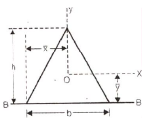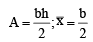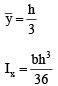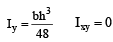(f) Trapezoid (Origin of axes at centroid).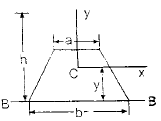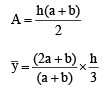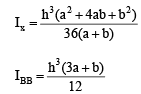(g) Circle (Origin of axes at center)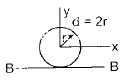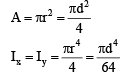(h) Quarter circle (Origin of axes at center of circle)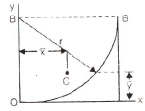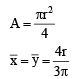4. Conjugate Beam Method

• This method is convenient if flexural rigidity of the beam is not uniform throughout the length of the beam. This method is suitable for beams carrying internal hinge. An imaginary beam for which the load diagram is (M/MI) diagram, of the given beam is called the conjugate beam.
(i) The slope at any section of the given beam is equal to the S.F. at the corresponding section of the conjugate beam i.e. SFD of conjugate beam is slope curve of real beam.
(ii) The deflection at any section for the given beam is equal to the bending moment at the corresponding section of the conjugate beam. i.e. BMD of conjugate beam is deflection curve of real beam.

Important points :
(i) A stable and statically determinate real beam will have a conjugate beam which is also stable and statically determinate.
(ii) A unstable real beam will have statically indeterminate conjugate beam, hence if a conjugate beam is found to be statically indeterminate, it is concluded that the real beam is unstable and further analysis is not appropriate.
(iii) Statically indeterminate real beam will have unstable conjugate beam hence its conjugate load must be such that it maintains equilibrium.
Summary of End Conditions of a Conjugate Beam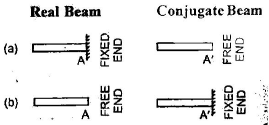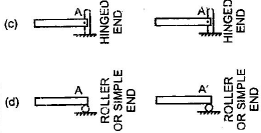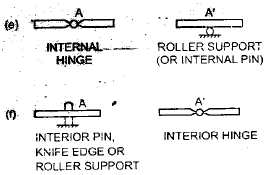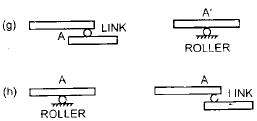5. Method of Superposition It is suitable for cantilevers containing concentrated loads & concentrated moments. This method can also be used for non prismatic bars i.e. varying EI(Flexural rigidity). 6. Strain Energy method (Castigliano’s theorem) It is suitable for cantilevers and beams having varying EI or varying depth of beam. This methods is very useful in case of determinate frames and arches. This can also be used when internal hinge is provided.

7. Unit load method

8. Dummy load method Method used to find deflection of truss joints (perfect frames) :
(i) Unit load method (Maxwell’s method)
(ii) Castigliano’s theorem (Strain energy method)
(iii) Graphical method (Williot Mohr diagram) (This method is used for trusses only & cannot be used for beams)

Offer running on EduRev: Apply code STAYHOME200 to get INR 200 off on our premium plan EduRev Infinity!

## Civil Engineering SSC JE (Technical)

113 docs|50 tests

,

,

,

,

,

,

,

,

,

,

,

,

,

,

,

,

,

,

,

,

,

;